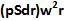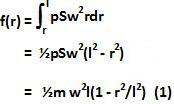## Wednesday, April 8, 2009

### Irodov Problem 1.296Consider an infinitesimally small piece of the rod at a distance r (point X) from the axis of rotation of length dr. If the density of the material is p and surface area of cross-section of the rod is S, then the mass of the infinitesimally small piece of rod will be pSdr. The centrifugal force experienced by this infinitesimally small piece will be.

Let the force with which the part of the rod XB pulls the part AX with a force f(r) given by,The stress is thus given by,The strain is thus given by,Suppose that the part XB was stretched bydue to the rotation, then we have,